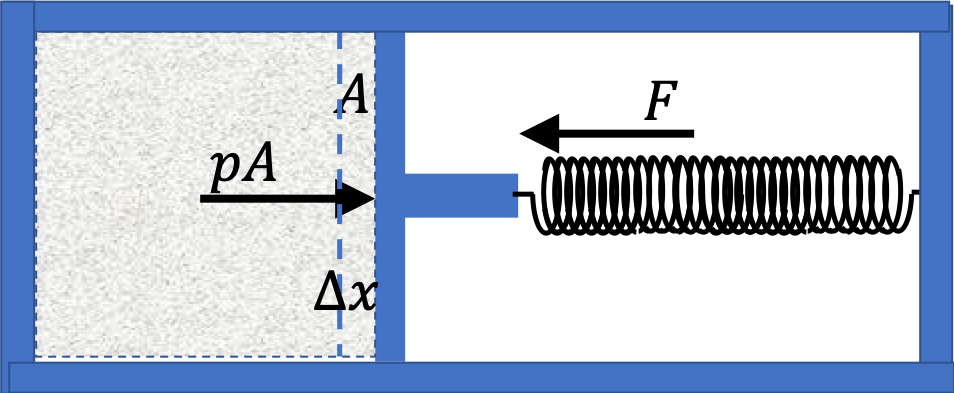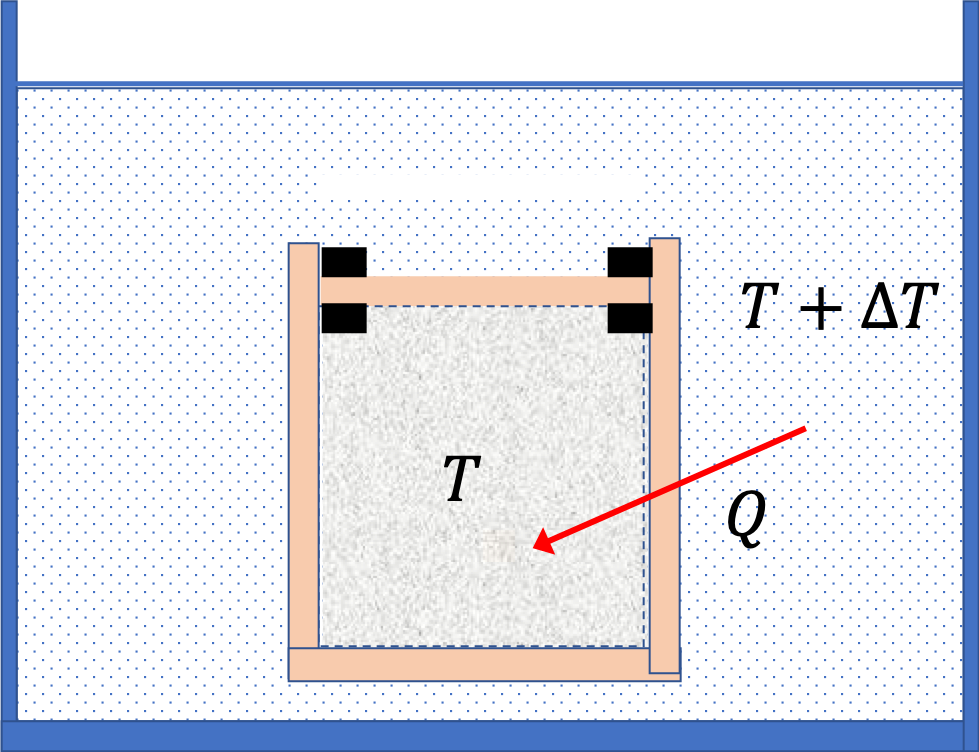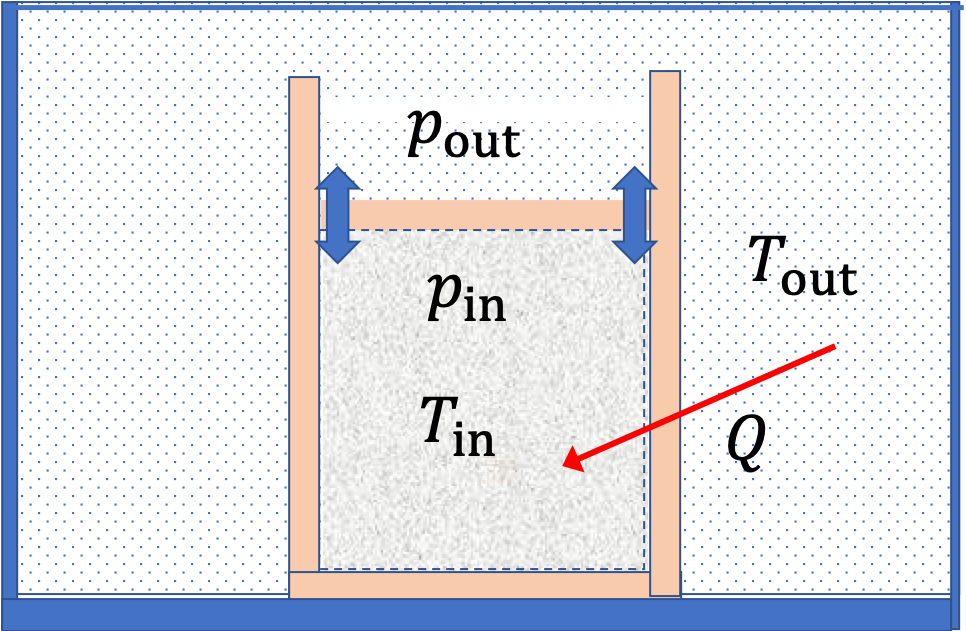## Section24.1First Law of Thermodynamics

### Subsection24.1.1Energy Change of a Thermally Insualted System

To get oriented with the energy considerations in a thermodynamic system, let us consider a simple system of a gas contained in a cylinder with movable piston as in Figure 24.1.1. Suppose the cylinder and piston are thermally insulated from the environment.Figure 24.1.1. The gas on the left side expands in a thermally insulated environment when piston moves against the spring. Energy is transferred between gas and spring.

Suppose the force on the piston by the spring is infinitesimally larger than the force by the gas so that the piston moves by a distance $\Delta x$ into the gas, corresponding to change in volume $\Delta V\text{.}$ The work done by the spring force will be

\begin{equation*} W_\text{by spring on gas} = -F\Delta x. \end{equation*}

During this time $F \approx pA$ and $\Delta V = A\Delta x \text{.}$ Thus, we can rewrite the work in state properties of the gas.

\begin{equation*} W_\text{by spring on gas} = -p\Delta V. \end{equation*}

The work by the gas will be opposite of this and so will have opposite sign.

\begin{equation} W_\text{by gas} = p\Delta V.\tag{24.1.1} \end{equation}

Thinking of gas as a thermodynamic system and the spring as its environment, we state this observation using the principle of the conservation of energy as follows.

\begin{equation*} \Delta E_\text{of gas} = -W_\text{by gas} = \text{Energy exchanged with environment.} \end{equation*}

Here, we see the exchange of energy occur through the performance of a mechanical work. Next we will see another mechanism of exchange of energy.

### Subsection24.1.2Energy Change of a Fixed-Volume System

In the above we saw how energy of a thermally insulated system can change by way of work. Let us now consider a situation as illustrated in Figure 24.1.2, where the system is in thermal contact with outside and no work by system is allowed by fixing its volume. To isolate the thermal effect, we examine a gas in a rigid container which is a good conductor of heat.Figure 24.1.2. The gas is heated by heating water in the bath and energy $Q$ flows into the gas through the thermally conductive walls. The gas is kept in fixed-volume enclosure so that the volume of the gas does not change and no work is done by the gas.

Suppose the gas is initially at temperature $T$ and in thermal equilibrium with a water bath also at temperature $T\text{.}$ When you heat water bath, its temperature will increase. You will find that energy begins to flow into the gas raising its temperature as well. But since the volume of the gas did not change, the energy change of the gas is not by a mechanical work, but by some other mechanism - a mechanism that requires a difference in temperarture across a thermally conductive material. This mechanism of energy transfer is what we have called heat transfer.

If you look at the heating process microscopically, you find that, as temperature of water rises, the water molecules move faster, and when they collide with the container, they transfer some of the energy to the container molecules, which in turn transfer energy to the gas molecules. The energy transfer by heat is the collective work of a large number of molecules.

We denote energy exchange by the mechanism of heat by letter $Q$ or $Q_\text{if}\text{,}$ where the subscript refers to the process, or $Q_\text{into}\text{,}$ where “into” is for energy entering into the system. Often $Q$ is called heat energy, but it is not like kinetic energy or potential energy, but rather an energy involved in transfer from one system, which is the water bath in our example, to another system, which is the gas, here.

\begin{equation*} \Delta E_\text{of gas} = Q_\text{into gas}. \end{equation*}

Once again, we can state this observation as a conservation of energy statment. Think of the gas as the thermodynamic system and the water bath as the environment, then we have

\begin{equation*} \Delta E_\text{gas} = Q_\text{into gas} = \text{Energy exchanged with environment.} \end{equation*}

Here, we saw the mechanism of exchange of energy was not through a mechanical work but through heat.

### Subsection24.1.3First Law of Thermodynamics

In the last two subsections, we identified two mechanisms by which energy of a thermodynamic system can change - (1) work $W$ done by the environment and (2) energy flow $Q$ due to a temperature difference across a thermal contact. Summing these two mechanisms gives us the statement of First Law of Thermodynamics.

Let us write the first law of thermodynamics in a more expressive language with appropriate subscripts.

\begin{equation} \Delta E_\text{of system} = Q_\text{into system} - W_\text{by system}.\label{eq-first-law-of-thermodynamics}\tag{24.1.2} \end{equation}

Notice that we need to be careful with the signs: $Q$ is positive if system heats up and negative if system cools, and $W$ is positive if system expands.

### Subsection24.1.4First Law of Thermodynamics and Internal Energy

Often in thermodynamics, we study energy changing processes that do not involve changing the translational and rotational kinetic energies or the gravitational potential energies or other forms of potential energy associated with the entire body.

The remaining energy is called internal energy. The internal energy is usually denoted by the symbol $U\text{,}$ which should not be confused with the same symbol used for the potential energy. The internal energy of a conservative system will be the sum of kinetic energies of all its molecules and the potential energies of interactions between the molecules.

\begin{equation} U = \sum_j K_j + \sum_{jk\text{-pairs}} U_{jk}.\tag{24.1.3} \end{equation}

In terms of internal energy, we write the first law of thermodynamics for the change in the energy of the system A as

\begin{equation} \Delta U = Q - W.\tag{24.1.4} \end{equation}

Sign Conventions:

1. Heat entering into the system: $Q \gt 0 \text{.}$
2. Heat leaving into the system: $Q \lt 0 \text{.}$
3. The system expanding: $W \gt 0 \text{.}$
4. The system contracting: $W \lt 0 \text{.}$

Consider the processes on a gas shown in Figure 24.1.4. In the process ab and bc, 3600 J and 2400 J of heat are added to the system. (a) Find the work done in each of the processes ab, bc, ad and dc. (b) Find the internal energy change in processes ab and bc. (c) Find the internal energy difference between states c and a. (d) Find the total heat added in the adc process. (e) From the information give, can you find heat added in process ad? Why or why not?

Hint

For work: use area under curve. For internal energy, use first law.

(a) - 810.4 J, (b) $\Delta U_{ab}$ = 3600 J, $\Delta U_{ab}$ = 374 J,(c) 3974 J, (d) 4784 J, and (e) Cannot be determined.

Solution

(a)

\begin{align*} \amp W_{ab} = 0,\\ \amp W_{bc} = 5\ \text{atm}\times 4\ \text{L} \times 101.3\ \frac{\text{J}}{\text{L.atm}} = 2,026\ \text{J},\\ \amp W_{ad} = 2\ \text{atm}\times 4\ \text{L} \times 101.3\ \frac{\text{J}}{\text{L.atm}} = 810.4\ \text{J}, \\ \amp W_{dc} = 0. \end{align*}

(b) For the a-b process:

\begin{equation*} \left. \begin{array}{l} W_{ab} = 0\\ Q_{ab} = 3,600\ \text{J} \end{array} \right\} \ \ \ U_{ab} = Q_{ab} - W_{ab} = 3,600\ \text{J}. \end{equation*}

For the b-c process:

\begin{equation*} \left. \begin{array}{l} W_{bc} = 2,026\ \text{J}\\ Q_{bc} = 2,400\ \text{J} \end{array} \right\} \ \ \ U_{bc} = Q_{bc} - W_{bc} = 374\ \text{J}. \end{equation*}

(c)

\begin{equation*} U_{ac} = U_{ab} + U_{bc} = 3,600 \ \text{J} + 374 \ \text{J}= 3,974\ \text{J}. \end{equation*}

(d)

\begin{align*} Q_{adc} \amp = U_{ac} + W_{ad} + W_{dc}\\ \amp = 3974 \ \text{J} + 810.4 \ \text{J} + 0 = 4,784.4 \ \text{J}. \end{align*}

(e) We cannot determine $Q_{ad}$ since we do not know $U_{ad}$ or have a way of determining it. We know only $W_{ad}\text{.}$ That would give

Consider the process for steam in a cylinder shown in Figure 24.1.6. Suppose the change in the internal energy in this process is 30 kJ. Find the heat entering the system.

Hint

Use area under the curve to find the work. Then, use first law.

$61,900\ \text{J}\text{.}$

Solution

The work by the system in the given process is

\begin{equation*} W_{ab} = \text{Area under the curve to the } p = 0 \text{ line.} \end{equation*}

This gives the sum of the area of a triangle and a rectangle.

\begin{align*} W_{ab} \amp = \left(\frac{1}{2} \times 9\ \text{L}\times 30\ \text{atm} + 9\ \text{L}\times 20\ \text{atm}\right) \times \frac{101.3\text{ J}}{\text{L.atm}}\\ \amp = 31,910\ \text{J}. \end{align*}

Therefore

\begin{equation*} U_{ab} = Q_{ab} + W_{ab}\ \ \Longrightarrow\ \ Q_{ab} = 61,900\ \text{J}. \end{equation*}

The state of 30 moles of steam in a cylinder is changed in a cyclic manner from a-b-c-a, where the pressure and volume of the states are a(20 L, 30 atm), b(20 L, 50 atm), and c(45 L, 50 atm), as shown in Figure 24.1.8. Assume each change takes place along the line connecting the initial and final states in the $PV$ plane. (a) Find the net work done by the steam in one cycle. (b) Find the net amount of heat flow in the steam over the course of one cycle.

Hint

(a) Note the work in a cycle is equal to the area enclosed in the PV plane. (b) Internal energy change is zero in a cyclic process.

(a) $25,300\ \textrm{J} \text{,}$ (b) $25,300\ \textrm{J}\text{.}$

Solution 1 (a)

(a) The net work will be sum of positive work in bc process and negative work on ca process. This sum equals the area enclosed in the cyclic process, which is just the area of a triangle.

\begin{equation*} W_{abca} = \frac{1}{2} \times 25\ \text{L}\times 20\ \text{atm} = 250\text{ L.atm} \end{equation*}

Converting this to Joules we get

\begin{equation*} W_{abca} = 250\text{ L.atm} \times \frac{101.3\ \textrm{J} }{\textrm{L.atm}} = 25,300\ \textrm{J}. \end{equation*}
Solution 2 (b)

(b) In a cycle, the state returns to the self. Therefore, the change in internal energy will be zero.

\begin{equation*} U_{abca} = 0. \end{equation*}

Now, we have the inernal energy change and work over one cylce. Therefore, we use the first law of thermodynamics to figure out heat exchanged.

\begin{equation*} Q_{abca} = U_{abca} + W_{abca} = 25,300\ \textrm{J}. \end{equation*}

### Subsection24.1.5Other Forms of Energy

Yet another approach to energy conservation is based on the various types of energy sources in common vernacular use. For instance, some chemical reactions release energy, we call that energy to be Chemical Energy. Similarly, the energy in a nuclear reaction is called the Nuclear Energy.

Classifying energy into various types is helpful in this regard since an overall energy of each type can often be determined from experiments. The change in internal energy is then written as a sum of

\begin{align*} \Delta U \amp = \Delta E_{\text{chem}} + \Delta E_{\text{nuc}} + \Delta E_{\text{atom}} + \cdots, \end{align*}

where various terms have the following interpretation.

1. Chemical energy, $E_{\text{chem}} \text{,}$
2. Nuclear energy, $E_{\text{nuc}} \text{,}$
3. Atomic random motion, $E_{\text{atom}} \text{,}$
4. Molecular rotational energy, $E_{\text{mol.rot.}} \text{,}$ etc.

### Subsection24.1.6Adiabatic Work and Internal Energy

Joule conducted experiments on the effect of stirring on the change in the state of water as determined from the change in the temperature of water. He found that, if the container is thermally insulated, the amount of work associated with the change of the state of water was independent of how the work was performed.

\begin{equation} W_{if} = U_f - U_i \ \ \ \text{(Adiabatic)}\tag{24.1.5} \end{equation}

This is what you expect from the first law of thermodynamics when $Q = 0\text{.}$ The work here is also called adiabatic work. The adiabatic work can be used to find internal energy of a system with respect to a reference state, called the standard state.

If we set the internal energy of the standard state as $U_0\text{,}$ then the internal energy of any arbitrary state A can be defined by appropriate adiabatic process.

\begin{equation} U_A = U_0 - W_{\text{standard-to-A}} \ \ \ \text{(in an adiabatic process)}\tag{24.1.6} \end{equation}

If no adaiabatic process can take you from the standard state to state A, you gan think of going from standard to some other state B and then from B to A, then we will get the internal energy at for state A by

\begin{equation} U_A = U_B - W_{\text{B-to-A}} = U_0 - W_{\text{standard-to-B}} - W_{\text{B-to-A}}.\tag{24.1.7} \end{equation}

This way, you can find the internal energy of all states of the system. We say that internal energy can be defined for every state and hence internal energy is said to be a state property.

### Subsection24.1.7(Calculus) Infinitesimal First law of thermodynamics

The first law of thermodynamics can be applied to any system in the universe. Suppose a system A interacts with the another system B such that in an infinitesimal time $dt$ an infinitesimal amount of heat $\delta Q$ flows into A and an infinitesimal amount of work $\delta W$ is done by A. Then, the first law of thermodynamics says that the internal energy of A will change by $dU\text{,}$

\begin{equation} dU = \delta Q - \delta W,\tag{24.1.8} \end{equation}

where I have used different symbols for infinitesimals for $U$ and for $Q$ and $W$ to indicate that while A has definite values of $U$ in each state of A since $U$ is a state function, $Q$ and $W$ are not state variables and A does not have any definite values of $Q$ and $W\text{.}$

This infinitesimal equation is applicable to all closed systems. In a hydrostatic system, such as gas in a tank, work is done as a result of change of volume. Therefore, in these systems we can write the energy involved in the work on the system in another form. This type of system is a very useful system for exploring quantitative implications of thermodynamic principles in significant detail. Many of the examples in this book will come from these systems.

To derive the form of the first law of thermodynamics in hydrostatic systems, consider a tank of gas in equilibrium with the environment. Let the tank have a freely movable piston and the conduct heat so that initially, say $t=0\text{,}$ the pressure $p_\text{in}$ inside the gas is equal to the pressure outside $p_\text{out}\text{,}$ and temperature $T_\text{in}$ inside the gas is equal to the temperature $T_\text{out}$ outside.We can denote the common pressure by $p$ and the common temperature by $T\text{.}$ Suppose we pull the piston outward very slowly so that the volume of the gas increases by amount $dV$ but the pressure in the gas is maintained at $p$ all the time.

The gas will do a work $-pdV$ and an infinitesimal amount of heat $dQ$ will enter the gas during this process. Therefore the change in internal energy of the gas for an infinitesimal process in this system will be given by the following relation.

\begin{equation} dU = dQ - pdV. \tag{24.1.9} \end{equation}
This is the infinitesimal form of the First Law of Thermoidynamics. For finite changes in state, this has to be integrated over the path of the process. Suppose, a process, changes the state of the gas from $(p_1, V_1)$ to $(p_2, V_2)\text{.}$ Then an integration over the path of the process will give us the change in internal energy.
\begin{equation} \Delta U = \int_{\text{path}}dQ - \int_{\text{path}}pdV.\tag{24.1.10} \end{equation}